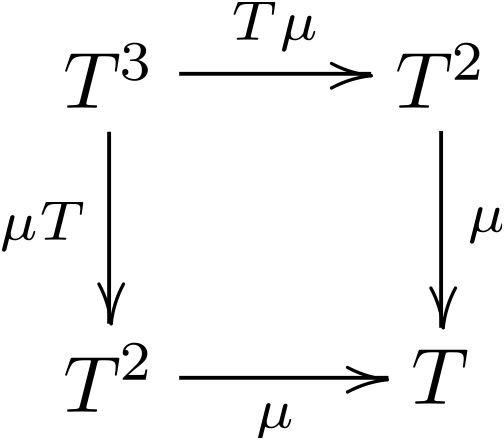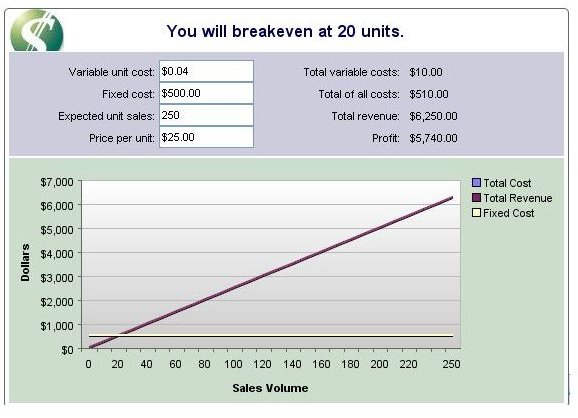# Learn Simple Examples of Calculating Fixed and Variable Costs

Page content

## Not All Costs Are Equal

Most business owners do understand a fixed cost from a variable cost. Fixed costs remain the same such as rent, mortgage payments, loans, payroll, etc. A variable cost is one that varies and includes items like sales commissions, labor, and cost of sales.

Even in the world of differentiating between fixed and variable costs, business analysts suggest that when calculating fixed and variable costs, your industry plays an important role. What is a variable cost for one industry may be a fixed cost for another.

So if all fixed and variable costs are not equal, what are some simple examples of calculating fixed and variable costs?

Because I have an auto dealership, I have many fixed and variable costs and most of the variable costs are departmental in nature such as sales commissions, vehicle inventory costs, policy adjustments (when my staff makes an error and I have to eat the expense), shipping, and labor.

Along with these variable costs in each department there are also fixed costs by department and fixed costs for the overall operation of the dealership. Fixed departmental costs are advertising, and certain salaried employees as well as inventory flooring. Fixed costs for the dealership, although they vary from month to month in amount, are office salaries, insurance, taxes, utilities, telephone, and office expense for example.

A small independent candle store or a drycleaner may not have certain variable costs as that industry is different.

## Calculating Fixed and Variable CostsBecause variable costs involve sales, production or manufacturing of a product or service, it’s important to learn simple examples for calculating fixed and variable costs to determine your breakeven analysis.

Let’s first look at calculating both fixed and variable costs. Here’s a simple example of calculating fixed and variable costs for a candle store:

• Calculating Variable Costs – Think of variable costs as anything that has to do with the production of your product or services. So, the calculation would be: Variable Costs = (cost per unit) divided (the number of units sold). If you sold candles and it cost you \$10 to manufacture each and expected unit sales are 250; your cost of production would be \$0.04 per unit or \$1,000 in variable costs.
• Calculating Fixed Costs – Fixed costs can be determined by simple addition. Once you determine your fixed costs, to obtain the total cost of your product or service, the calculation is total fixed costs plus your variable costs. Say your fixed costs per any given month are \$500 and we already know from the example above that are variable cost is \$0.04 per unit or \$1,000. Total costs would equal both your fixed and variable costs; or \$1,000 (variable) and \$500 (fixed) for total costs of \$1,500.

Now that you know the simple formulas for determining fixed and variable costs, what about your breakeven analysis?

It’s important to note that to determine your breakeven analysis, you must consider the price of the product and the formula for determining breakeven is (Fixed Costs divided by Unit Selling Price, less Variable Costs).

Continuing on with our example above, if we sold each candle for \$25 and we know we sold 250 that would mean \$6,250 in revenues.If you look at the image to the bottom left of a breakeven analysis calculator courtesy of KJE Computer Solutions (click to enlarge), you will see from our numbers above, the candle company would have to sell 20 units to breakeven. A higher percentage of sales would realize a profit.

## Why Are These Calculations So Important?

Part of starting, managing, and running a business means knowing how to determine your breakeven analysis by using simple examples of determining fixed and variable costs. In our example, if we had sold 30 units instead of the 20 needed to breakeven, we’d have an additional profit and would be able to handle both fixed and variable expenses as well as overhead costs.

These formulas are also great tools for sales and expense forecasting to help you forecast numbers that are more accurate.

Every business owner should implement these formulas for determining fixed and variable costs and when asked, should know his or her breakeven analysis at any given time. Guessing how many products you need to produce and what price you need to set is not a wise choice when attempting to figure out how high your sales revenues must be to realize a profit.

## Other Considerations for Fixed & Variable Costs

As stated earlier, you must let the type of industry you are in guide you when determining the difference between fixed and variable costs. There can also be mixed costs, which are costs that may fit into both categories.

To determine your breakeven analysis, if you use these simple examples of fixed and variable costs, along with an effective breakeven analysis calculator, you will know where your business stands as far as expected sales, expenses and profits (or losses).